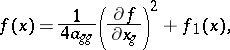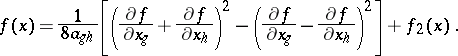# Lagrange method

Jump to: navigation, search

A method for reducing a quadratic form (cf. also Quadratic forms, reduction of) to a sum of squares, given by J.L. Lagrange in 1759. Suppose one is given a quadratic form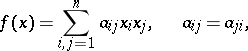(1)

invariables, with coefficients from a fieldof characteristic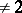. It is required to reduce this form to the canonical form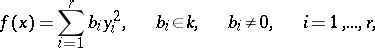(2)

by means of a non-singular linear transformation of the variables. Lagrange's method consists in the following. One may assume that not all the coefficients in (1) are zero. Therefore, two cases are possible.

1) For some,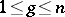, the diagonal coefficient. Then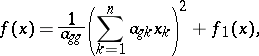(3)

where the form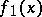does not contain the variable.

2) If all the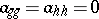, but, then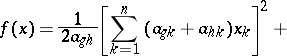(4)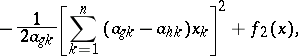where the form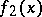does not contain the two variablesand. The forms in square brackets in (4) are linearly independent. By using transformations of the form (3) and (4), after finitely many steps one can reduce the form (1) to a sum of squares of linearly independent linear forms. By means of partial derivatives the formulas (3) and (4) can be written as